# GMAT Math : DSQ: Solving quadratic equations

## Example Questions

### Example Question #1 : Solving Quadratic Equations

Consider the equation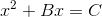How many real solutions does this equation have?

Statement 1: There exists two different real numbers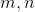such that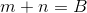and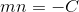Statement 2:is a positive integer.

Statement 2 ALONE is sufficient to answer the question, but Statement 1 ALONE is NOT sufficient to answer the question.

Statement 1 ALONE is sufficient to answer the question, but Statement 2 ALONE is NOT sufficient to answer the question.

BOTH statements TOGETHER are sufficient to answer the question, but NEITHER statement ALONE is sufficient to answer the question.

EITHER statement ALONE is sufficient to answer the question.

BOTH statements TOGETHER are insufficient to answer the question.

EITHER statement ALONE is sufficient to answer the question.

Explanation:can be rewritten as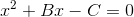If Statement 1 holds, then the equation can be rewritten as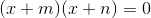. This equation has solution set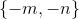, which comprises two real numbers.

If Statement 2 holds, the discriminant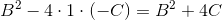is positive, being the sum of a nonnegative number and a positive number; this makes the solution set one with two real numbers.

### Example Question #2 : Solving Quadratic Equations

Let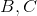be two positive integers. How many real solutions does the equation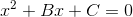have?

Statement 1:is a perfect square of an integer.

Statement 2: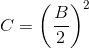BOTH statements TOGETHER are insufficient to answer the question.

BOTH statements TOGETHER are sufficient to answer the question, but NEITHER statement ALONE is sufficient to answer the question.

Statement 1 ALONE is sufficient to answer the question, but Statement 2 ALONE is NOT sufficient to answer the question.

EITHER statement ALONE is sufficient to answer the question.

Statement 2 ALONE is sufficient to answer the question, but Statement 1 ALONE is NOT sufficient to answer the question.

Statement 2 ALONE is sufficient to answer the question, but Statement 1 ALONE is NOT sufficient to answer the question.

Explanation:

The number of real solutions of the equation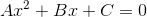depends on whether discriminantis positive, zero, or negative; since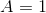, this becomes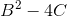.

If we only know thatis a perfect square, then we still need to knowto find the number of real solutions. For example, let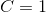, a perfect square. Then the discriminant is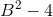, which can be positive, zero, or negative depending on.

But if we know, then the discriminant is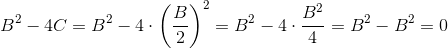Therefore,has one real solution.

### Example Question #3 : Solving Quadratic Equations

Does the solution set of the following quadratic equation comprise two real solutions, one real solution, or one imaginary solution?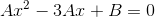Statement 1: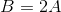Statement 2: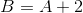BOTH statements TOGETHER are insufficient to answer the question.

BOTH statements TOGETHER are sufficient to answer the question, but NEITHER statement ALONE is sufficient to answer the question.

Statement 1 ALONE is sufficient to answer the question, but Statement 2 ALONE is NOT sufficient to answer the question.

EITHER statement ALONE is sufficient to answer the question.

Statement 2 ALONE is sufficient to answer the question, but Statement 1 ALONE is NOT sufficient to answer the question.

Statement 1 ALONE is sufficient to answer the question, but Statement 2 ALONE is NOT sufficient to answer the question.

Explanation:

The sign of the discriminant of the quadratic expression answers this question; here, the discriminant is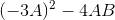,

or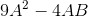If we assume Statement 1 alone, this expression becomes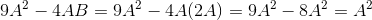Since we can assumeis nonzero,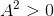. This makes the discriminant positive, proving that there are two real solutions.

If we assume Statement 2 alone, this expression becomes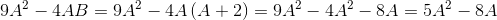The sign of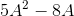can vary.

Case 1:Then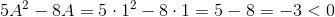giving the equation two imaginary solutions.

Case 2: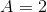Then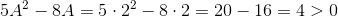giving the equation two real solutions.

Therefore, Statement 1, but not Statement 2, is enough to answer the question.

### Example Question #4 : Solving Quadratic Equationsis a positive integer.

True or false: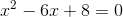Statement 1:is an even integer

Statement 2: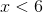Statement 2 ALONE is sufficient to answer the question, but Statement 1 ALONE is NOT sufficient to answer the question.

BOTH statements TOGETHER are sufficient to answer the question, but NEITHER statement ALONE is sufficient to answer the question.

Statement 1 ALONE is sufficient to answer the question, but Statement 2 ALONE is NOT sufficient to answer the question.

EITHER statement ALONE is sufficient to answer the question.

BOTH statements TOGETHER are insufficient to answer the question.

BOTH statements TOGETHER are sufficient to answer the question, but NEITHER statement ALONE is sufficient to answer the question.

Explanation:

The quadratic expression can be factored as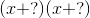, replacing the question marks with integers whose product is 8 and whose sum is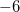. These integers are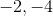, so the equation becomes: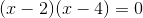Set each linear binomial to 0 and solve: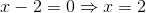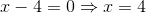Therefore, for the statement to be true, eitheror. Each of Statement 1 and Statement 2, taken alone, leaves other possible values of. Taken together, however, they are enough, since the only two positive even integers less than 6 are 2 and 4.

### Example Question #5 : Solving Quadratic Equationsis a positive integer.

True or false?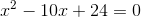Statement 1:is an even integer

Statement 2: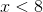BOTH statements TOGETHER are insufficient to answer the question.

Statement 1 ALONE is sufficient to answer the question, but Statement 2 ALONE is NOT sufficient to answer the question.

EITHER statement ALONE is sufficient to answer the question.

Statement 2 ALONE is sufficient to answer the question, but Statement 1 ALONE is NOT sufficient to answer the question.

BOTH statements TOGETHER are sufficient to answer the question, but NEITHER statement ALONE is sufficient to answer the question.

BOTH statements TOGETHER are insufficient to answer the question.

Explanation:

The two statements together are insufficient. If both are assumed, thencan be 2, 4, or 6.

Ifthe statement is true: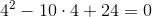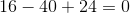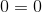But ifthe statement is false: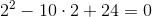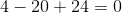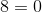### Example Question #6 : Solving Quadratic Equations

What are the solutions of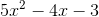in the most simplified form?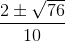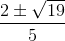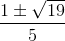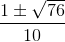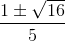Explanation:

This is a quadratic formula problem. Use equation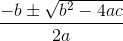. For our problem,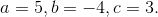Plug these values into the equation, and simplify: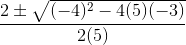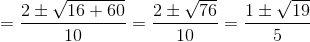. Here, we simplified the radical by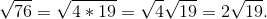Tired of practice problems?

Try live online GMAT prep today.